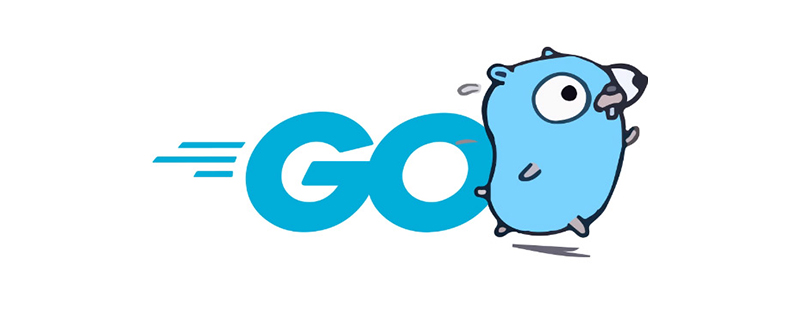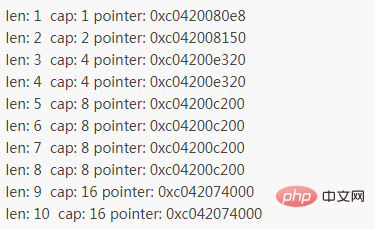# go语言中切片怎么加元素Go语言 append()函数

append可以向一个slice中追加一个元素、多个元素、新的切片

```var a []int
a = append(a, 1) // 追加1个元素
a = append(a, 1, 2, 3) // 追加多个元素, 手写解包方式
a = append(a, []int{1,2,3}...) // 追加一个切片, 切片需要解包```

```var numbers []int

for i := 0; i < 10; i++ {
numbers = append(numbers, i)
fmt.Printf("len: %d  cap: %d pointer: %p\n", len(numbers), cap(numbers), numbers)
}```• 第 1 行，声明一个整型切片。

• 第 4 行，循环向 numbers 切片中添加 10 个数。

• 第 5 行，打印输出切片的长度、容量和指针变化，使用函数 len() 查看切片拥有的元素个数，使用函数 cap() 查看切片的容量情况。

• 员工和工位就是切片中的元素。

• 办公地就是分配好的内存。

• 搬家就是重新分配内存。

• 无论搬多少次家，公司名称始终不会变，代表外部使用切片的变量名不会修改。

• 由于搬家后地址发生变化，因此内存“地址”也会有修改。

```var a = []int{1,2,3}
a = append([]int{0}, a...) // 在开头添加1个元素
a = append([]int{-3,-2,-1}, a...) // 在开头添加1个切片```

```var a []int
a = append(a[:i], append([]int{x}, a[i:]...)...) // 在第i个位置插入x
a = append(a[:i], append([]int{1,2,3}, a[i:]...)...) // 在第i个位置插入切片```

【相关推荐：Go视频教程编程教学#### 相关文章推荐

• 聊聊go语言中的切片（slice）• golang中切片是啥• 一文浅析Golang中的切片（Slice）• go语言怎么从切片中删除元素• go语言中切片和数组是什么
1/1